# Bauer-Peschl equation

A second-order elliptic partial differential equation in(if) or in the open unit disc(if), of the form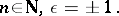By using,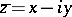,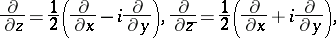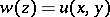, one arrives at the standard notation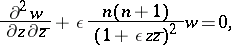(a1)This equation attracted interest from the differential-geometric viewpoint from the times of G. Darboux and H.A. Schwarz. It played an important role in the investigation of differential invariants for certain families of complex functions by E. Peschl ([a8], [a9]) and has been treated systematically by a number of authors since then. In particular, the papers of K.W. Bauer [a2], [a1] have stimulated further investigations and have been significant. Summaries of the results, including the essential contributions of S. Ruscheweyh, M. Kracht and E. Kreyszig can be found in [a3] and in [a7].

For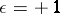, equation (a1) transforms by stereographic projection into the equation of spherical harmonics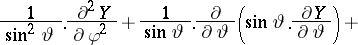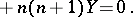Separation of variables then readily shows the connection of (a1) with the equations of mathematical physics (cf. also Mathematical physics, equations of), to wit, the-dimensional potential equation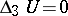and the-dimensional wave equation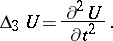Analogously, for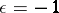equation (a1) is connected to the equation of hyperboloid functions and tothe-dimensional wave equation (see [a2], [a1]). Moreover, (a1) is related to the generalized axially symmetric potential theory, the theory of poly-analytic and poly-harmonic functions, the theory of Eisenstein series, etc. (see [a7]).

Motivated by these relations, K.W. Bauer has given detailed representations of the solutions of (a1) by using differential operators:whereand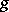may be arbitrary holomorphic functions (cf. also Holomorphic function). This kind of representation of solutions can be transferred to certain more general equations than (a1), similar to the integral representations of S. Bergman and I.N. Vekua, and facilitates the creation of function theories associated with the classical theory of holomorphic functions (see [a2], [a1], [a3], [a4], [a5], [a6], [a7]). This allows the treatment of classical problems (e.g. the Riemann–Hilbert problem, the Cauchy–Kovalevskaya problem, cf. also Cauchy–Kovalevskaya theorem, etc.) for these classes of functions.

How to Cite This Entry:
Bauer-Peschl equation. Encyclopedia of Mathematics. URL: http://encyclopediaofmath.org/index.php?title=Bauer-Peschl_equation&oldid=40961
This article was adapted from an original article by R. Heersink (originator), which appeared in Encyclopedia of Mathematics - ISBN 1402006098. See original article